Mathematics - All About Rational Expressions

in STEMGeeks2 months ago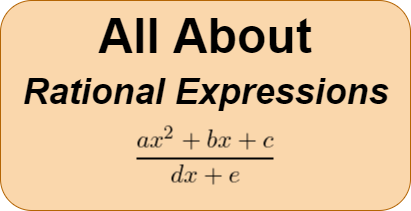Introduction

Hey it's a me again @drifter1!

Today's article is another high-school refresher on Mathematics, and more specifically on Rational Expressions. It's a follow-up to the previous post on Polynomial Arithmetic. Rational Expressions are basically a quotient of polynomials, thus knowledge on them is quite important for this article.

So, without further ado, let's get straight into it!

Rational Expressions

A rational expression is simply a fraction with polynomials, and in its simplest form can be thought of as the "ratio" of two polynomials. The polynomial over the line is the numerator, whilst the polynomial below the line is the denominator.

For example, the following are valid rational expressions: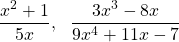whilst the following are not: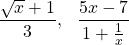because the numerator in the first case, and the denominator in the second case, are not valid polynomials.

Of course the denominator can't be zero, therefore a more general representation is: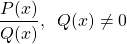where P(x), Q(x) are two valid polynomials.

Proper - Improper

In fractions, if the numerator is larger than the denominator, the fraction is improper. Otherwise, it's called proper. Similarly, if the degree of the numerator polynomial is higher than or equal to the degree of the denominator polynomial, the rational expression is given the name improper. Such a rational expression can mostly be simplified using Polynomial Division.

For example, the following is an improper rational expression: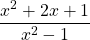which can be simplified as follows: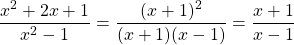using the two most commonly known formulas.

Function Domain

Of course, when talking about functions its important to understand that the Domain of the original function might not be the same as the one in the simplified result.

In the previous example, the original function's Domain doesn't include both 1 and -1, because the denominator would be zero making the rational expression undefined. But, in the simplified result, the Domain doesn't include 1. As such, the two completely different functions!

Roots of Rational Expressions

We haven't covered finding roots of polynomials (which is an interesting topic for a later article though!), but finding the roots of a rational expression is the same as finding the roots of the numerator or top polynomial. The only other condition to be met for that to be true is that the rational expression needs to be in Lowest Terms.

Lowest Terms basically means that the top and bottom polynomials have no common factors.

In the context of fractions, it's easier to spot. For example, 3 / 6 has a common factor of 3, and the lowest terms form is 1 / 2.

Similarly, the original rational expression from before was not in lowest terms, as there was a common factor of (x + 1). But, the result of simplifcation is in lowest terms as x + 1 and x - 1 have no common factors, because x + 1 was cancelled out.

Multiplication

Multiplying two rational expressions is as simple as multiplying the tops and bottoms separately, in order to end up with the result.

For example: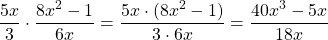Using this concept it's possible to multiply the top and bottom with a simple polynomial of the form (x + a) in order to simplify either of the two polynomials further. But, of course the Domain of the original rational expression should be taken into account!

Division

Dividing two Rational Expressions is as simple as flipping the second one (turning it into the reciprocal) and then multiplying as shown previously. You can also think of it as multiplying the outer and inner polynomials: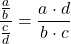For example:It's funny that addition and subtraction comes so late, but it depends on the previous ones. The easiest way of adding and subtracting is using the common denominator method, which looks like this: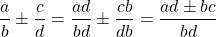In other words, in order to end up with the same denominator, the first rational expression is multiplied top-bottom by the second's denominator, and the second rational expression is multiplied top-bottom by the first's denominator. Afterwards, the numerators can be added together and the result be simplified as needed.

For example: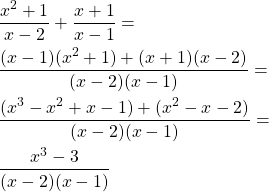Complex Fractions

If the numerator and / or denominator of a fraction is an rational expression or any sum, difference etc. of rational expressions, we talk about an complex fraction.

In that case the numerator and denominator need to be turned into a single rational expression. And as a follow-up the division of these two rational expressions is calculated using the technique shown previously.

For example: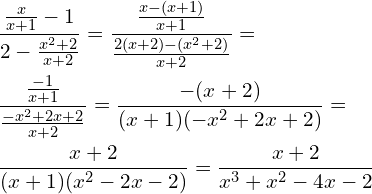RESOURCES:

References

Block diagrams and other visualizations were made using draw.io.

Final words | Next up

And this is actually it for today's post!